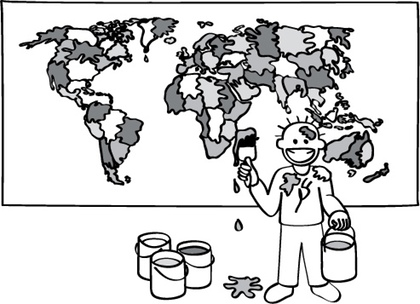Graph Colouring

The Poor Cartographer #

Coloring a map (which is equivalent to a graph) sounds like a simple task, but in computer science this problem epitomizes a major area of research looking for solutions to problems that are easy to make up, but seem to require an intractable amount of time to solve.

This activity introduces graph colouring, and leads on to many variations and extensions that reach the cutting edge of computer science.Activity description (PDF) #

If you want to find out more #

• Wikipedia: Graph Coloring
• Wikipedia: Graph Theory
• Wikipedia: Glossary of Graph Theory
• Wikipedia: Matching (Graph Theory) – In the mathematical discipline of graph theory, a matching or independent edge set in a graph is a set of edges without common vertices. It may also be an entire graph consisting of edges without common vertices
• Some Other Intractable Problems: Graph colouring is just one of thousands of intractable problems, many of which have confounded scientists for decades. Here are a couple more examples:
• Travelling Salesperson Problem – Given a collection of cities connected by roads of different lengths, find the shortest possible path allowing for a ‘travelling salesperson’ to visit each city, before returning to their starting point. This may seem like a simple task, but the number of possible routes increases surprisingly quickly, leading to huge numbers with a relatively small amount of cities. Computer scientists have been pondering solutions to the TSP for over 50 years. In fact, there’s still a \$1,000,000 prize up for grabs for an effective solution. Georgia Tech has a number of available TSP resources, including games and real-life examples.
• Chess – Calculating a solution to a game of chess is not an intractable problem itself. In fact, computers have been beating humans at chess for over a decade. However, trying to calculate all of the possible paths to a solution leads to a combinatorial explosion, and demonstrates the limits of computer processing. A few other popular games that present intractable problems are Battleship, Minesweeper, and Sudoku.

• Computation